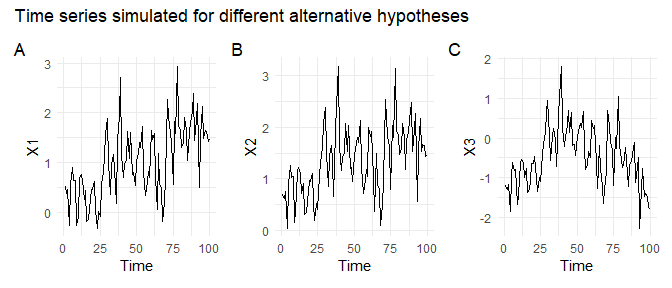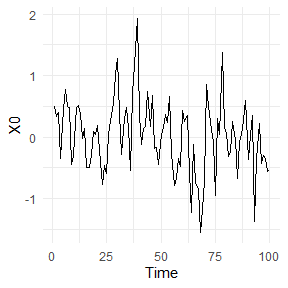# 1 Introduction

The majority of studies focus on detection of linear or monotonic trends, using

• classical t-test (for linear trends) or
• rank-based Mann–Kendall test (for monotonic trends)

typically under the assumption of uncorrelated data.

There exist two main problems:

• dependence effect, that is, the issue of inflating significance due to dependent observations when the test is developed for independent data (always check assumptions of the testing method!), and
• change points or regime shifts that affect the linear or monotonic trend hypothesis. For example, when testing the null hypothesis ($$H_0$$) of no trend against the alternative hypothesis ($$H_1$$) of linear trend, using t-test, it is easier to reject $$H_0$$ and accept $$H_1$$ in case A below, than in case B, and especially hard to reject $$H_0$$ in case C. Case C reminds us that a test with proper alternative hypothesis should be chosen, and that non-rejection of $$H_0$$ does not mean it is true.

These problems can be addressed by using tests for non-monotonic trends assuming that observations can be autocorrelated.

set.seed(777)
n <- 100
Time <- c(1:n)
X0 <- arima.sim(list(order = c(1, 0, 0), ar = 0.5), n = n, n.start = 100, sd = 0.5)
X1 <- 2*Time/n + X0
X2 <- 2*(Time/n)^0.5 + X0
X3 <- 0.5*(Time - n/2)/n - 6*((Time - n/2)/n)^2 + X0
X <- as.data.frame(cbind(X0, X1, X2, X3))
# Warning: package 'ggplot2' was built under R version 4.2.3The time series above were simulated:
A) X1 with linear trend,
B) X2 with square root – nonlinear monotonic – trend, and
C) X3 with quadratic – nonlinear non-monotonic – trend,
with stationary autocorrelated innovations X0: $$X0_t = 0.5X0_{t-1} + e_t$$, where $$e_t \sim N(0, 0.5^2)$$.Let’s test these time series using the functions from package funtimes, using significance level $$\alpha = 0.05$$.

To install and load the package, run

install.packages("funtimes")
library(funtimes)

# 2 Testing for presence of a trend

Function notrend_test tests the null hypothesis of no trend against different alternatives defined by the corresponding tests.

## 2.1 Linear trend

Consider the following pair of hypotheses
$$H_0$$: no trend
$$H_1$$: linear trend
that can be tested specifically using t-test.

Assuming the time series may be autocorrelated (which is the usual case with observational data), we apply sieve-bootstrap version of the t-test, by adapting the approach of Noguchi, Gel, and Duguay (2011):

notrend_test(X0)
#
#   Sieve-bootstrap Student's t-test for a linear trend
#
# data:  X0
# Student's t value = -2.6429, p-value = 0.098
# alternative hypothesis: linear trend.
# sample estimates:
# $AR_order #  1 # #$AR_coefficients
#     phi_1
# 0.4212756

The large $$p$$-value correctly indicates that there is not enough evidence to reject the hypothesis of no trend in X0 in favor of the alternative hypothesis of a linear trend.

For the other time series, $$p$$-values are reported below:

apply(X[,-1], 2, function(x) notrend_test(x)$p.value) # X1 X2 X3 # 0.000 0.002 0.858 indicating that the null hypothesis of no trend could be rejected and hypothesis of a linear trend could be accepted for X1 and X2. While X3 has a trend (based on the way it was simulated and the time series plot above), the alternative hypothesis of a linear trend does not fit in this case, so the test for linear trend (t-test) failed to reject the null hypothesis. ## 2.2 Monotonic trend Since a linear trend is also a monotonic trend, we may expect seeing similar results when testing the following pair of hypotheses $$H_0$$: no trend $$H_1$$: monotonic trend using Mann–Kendall test. Apply Mann–Kendall test, also with the sieve-bootstrap enhancement for potentially autocorrelated data; $$p$$-values are shown below: apply(X, 2, function(x) notrend_test(x, test = "MK")$p.value)
#    X0    X1    X2    X3
# 0.057 0.000 0.000 0.929

indicating that the null hypothesis of no trend could be rejected and hypothesis of a monotonic trend could be accepted for X1 and X2. For X0 and X3, the null hypothesis could not be rejected, because X0 does not have a trend, and X3 has a trend that does not match the alternative hypothesis.

## 2.3 Any trend

If the interest is in testing for any, potentially non-monotonic trend, consider testing the following pair of hypotheses
$$H_0$$: no trend
$$H_1$$: any trend
using local regression-based WAVK test (Wang, Akritas, and Van Keilegom 2008).

Apply WAVK test, also with the sieve-bootstrap enhancement for potentially autocorrelated data:

apply(X, 2, function(x) notrend_test(x, test = "WAVK",
factor.length = "adaptive.selection")$p.value) # X0 X1 X2 X3 # 0.337 0.000 0.026 0.004 The results indicate that WAVK test was correct in non-rejecting the null hypothesis for X0, and correctly rejected it for the time series with trends X1, X2, and X3. Lyubchich, Gel, and El-Shaarawi (2013) originally implemented hybrid bootstrap to this test statistic, available from the wavk_test function described in the next section. # 3 Testing a specific parametric form of trend Function wavk_test is developed for the following goodness-of-fit question (Lyubchich, Gel, and El-Shaarawi 2013): $$H_0$$: trend is of form $$f(\theta,t)$$ $$H_1$$: trend is not of form $$f(\theta,t)$$ where $$f$$ belongs to a known family of smooth parametric functions, and $$\theta$$ are its parameters. Note Considering $$f(\theta,t)$$ being some polynomial function, non-rejection of the null hypothesis means that function $$f(\theta,t)$$ or its simpler form (lower-order polynomial) is sufficient for describing the trend in the tested time series. Note The case of $$f(\theta,t) \equiv 0$$ corresponds to testing for no trend (in other words, for a constant trend, same as in the previous section), and the following code differs only in the type of bootstrap used, • sieve bootstrap in notrend_test (WAVK statistic is calculated on original time series and simulated autoregressive series) and • hybrid bootstrap in wavk_test (WAVK statistic is calculated on time series after the trend $$f(\theta,t)$$ and autoregressive dependence are removed, and on simulated independent normal series) notrend_test(X0, test = "WAVK", factor.length = "adaptive.selection") # WAVK with sieve bootstrap # # Sieve-bootstrap WAVK trend test # # data: X0 # WAVK test statistic = 8.7024, moving window = 4, p-value = 0.37 # alternative hypothesis: (non-)monotonic trend. # sample estimates: #$AR_order
#  1
#
# $AR_coefficients # phi_1 # 0.4212756 wavk_test(X0 ~ 0, factor.length = "adaptive.selection") # WAVK with hybrid bootstrap # # Trend test by Wang, Akritas, and Van Keilegom (bootstrap p-values) # # data: X0 # WAVK test statistic = 0.30965, adaptively selected window = 4, p-value # = 0.632 # alternative hypothesis: trend is not of the form X0 ~ 0. To test a linear trend $$f(\theta,t) = \theta_0 + \theta_1 t$$, use wavk_test(X0 ~ t, factor.length = "adaptive.selection") # # Trend test by Wang, Akritas, and Van Keilegom (bootstrap p-values) # # data: X0 # WAVK test statistic = -0.085378, adaptively selected window = 4, # p-value = 0.98 # alternative hypothesis: trend is not of the form X0 ~ t. Note that the time sequence t is specified automatically within the function. For the other time series, $$p$$-values are shown below: apply(X[,-1], 2, function(x) wavk_test(x ~ t, factor.length = "adaptive.selection")$p.value)
#    X1    X2    X3
# 0.954 0.786 0.020

The function poly could also be used, for example, test quadratic trend $$f(\theta,t) = \theta_0 + \theta_1 t + \theta_2 t^2$$ and show the trend estimates using the argument out = TRUE:

wavk_test(X3 ~ poly(t, 2), factor.length = "adaptive.selection", out = TRUE)
#
#   Trend test by Wang, Akritas, and Van Keilegom (bootstrap p-values)
#
# data:  X3
# WAVK test statistic = -0.097613, adaptively selected window = 4,
# p-value = 0.896
# alternative hypothesis: trend is not of the form X3 ~ poly(t, 2).
# sample estimates:
# $trend_coefficients # (Intercept) poly(t, 2)1 poly(t, 2)2 # -0.4860421 -0.2358495 -4.7102192 # #$AR_order
#  1
#
# $AR_coefficients # phi_1 # 0.4193298 # #$all_considered_windows
#  Window WAVK-statistic p-value
#       4    -0.09761277   0.896
#       5    -0.47737630   0.816
#       7    -0.47880434   0.860
#      10    -0.12694875   0.780

# Citation

This vignette belongs to R package funtimes. If you wish to cite this page, please cite the package:

citation("funtimes")
#
# To cite package 'funtimes' in publications use:
#
#   Lyubchich V, Gel Y, Vishwakarma S (2023). _funtimes: Functions for
#   Time Series Analysis_. R package version 9.1.
#
# A BibTeX entry for LaTeX users is
#
#   @Manual{,
#     title = {funtimes: Functions for Time Series Analysis},
#     author = {Vyacheslav Lyubchich and Yulia R. Gel and Srishti Vishwakarma},
#     year = {2023},
#     note = {R package version 9.1},
#   }